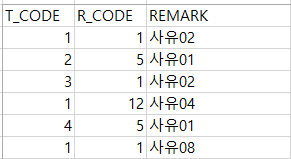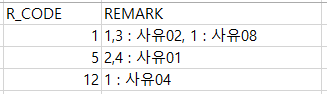## Mssql 쿼리 좀 도와주세요 Stuff for xml 관련..위의 데이터를 아래와 같이 나타내려고 하는데요

R_CODE를 그룹으로 T_CODE와 REMARK를 합친 내용입니다.

일반적으로 쓰는 제가 쓰는 For xml 으로는

T_CODE와 REMARK가 합쳐지지 않아서 도움 요청합니다

답변달아주시면 감사하겠슴다~

0
• ### 답변 1

• WITH T AS (
Select '1' as R_CODE, '1' as T_CODE, '사유02' as Remark union all
Select '5' as R_CODE, '2' as T_CODE, '사유01' as Remark union all
Select '1' as R_CODE, '3' as T_CODE, '사유02' as Remark union all
Select '12' as R_CODE, '1' as T_CODE, '사유04' as Remark union all
Select '5' as R_CODE, '4' as T_CODE,  '사유01' as Remark union all
Select '1' as R_CODE, '1' as T_CODE,  '사유08' as Remark
)

Select  R_CODE, STRING_AGG(T_CODE + ' : ' + Remark, ', ')
From (
Select  R_CODE, Remark, STRING_AGG(T_CODE, ',') WITHIN GROUP (Order by T_CODE) as T_CODE
From    t
Group by R_CODE, Remark
) as a
Group by R_CODE
Order by CAST(R_CODE as INT)